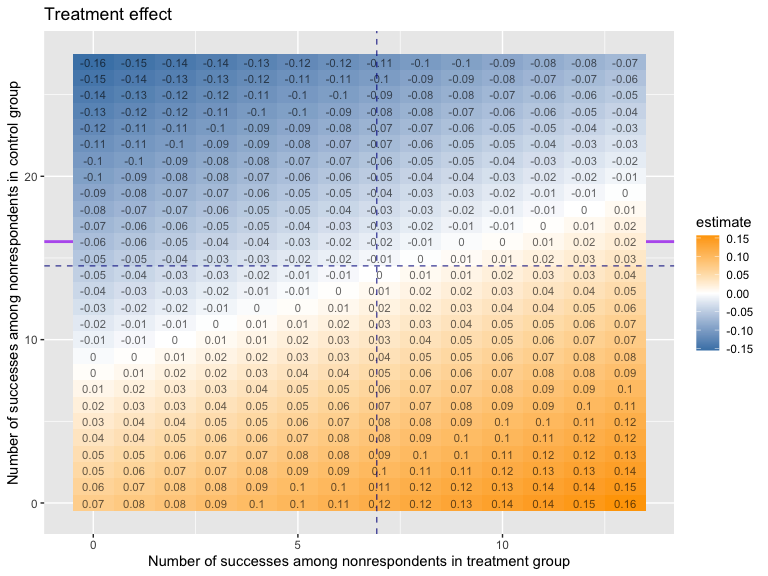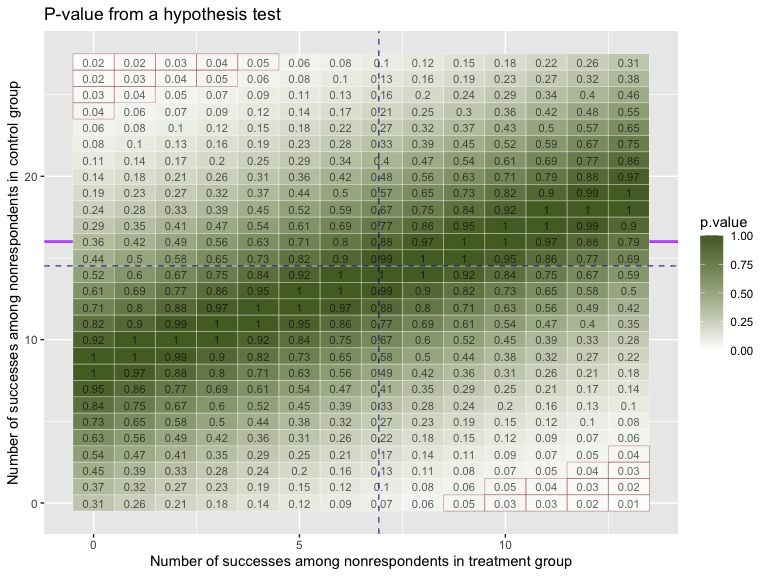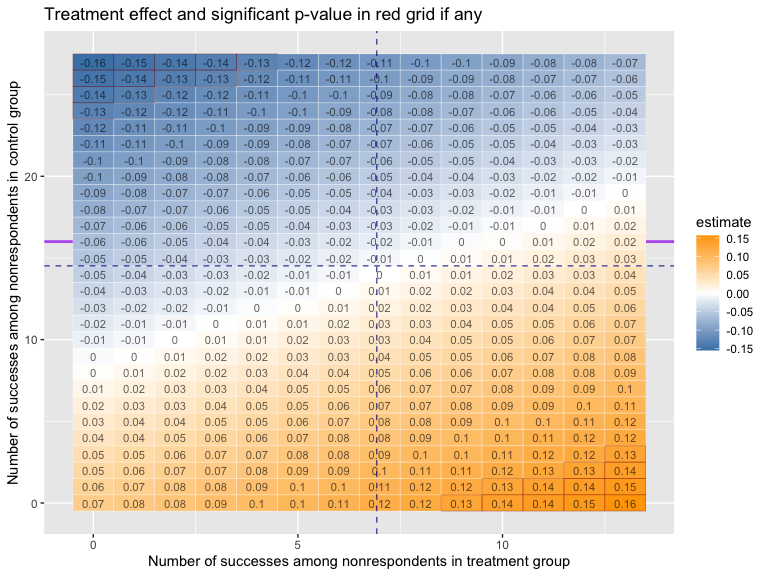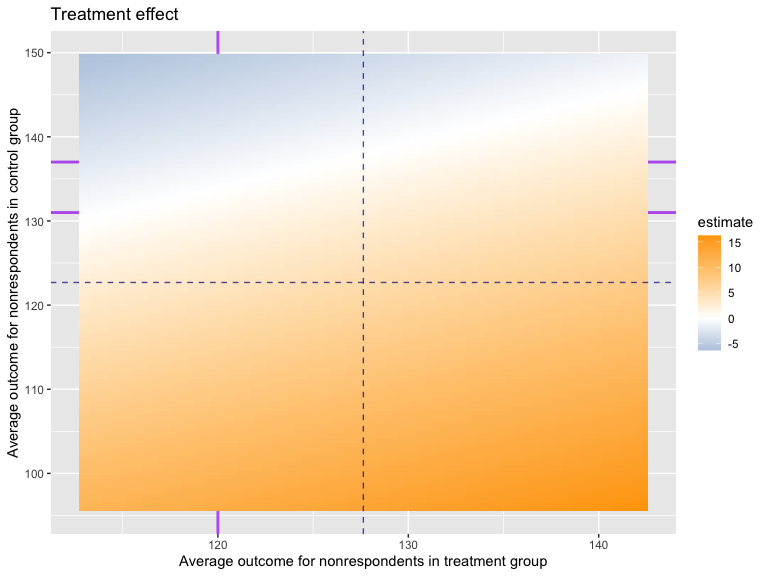# TippingPoint

A manual to show the R package `TippingPoint`.

## Introduction

The `TippingPoint` package aims to handle missing outcome data by listing out all the possible combinations of missing values in two treatment arms, calculating the corresponding estimated treatment effects and p-values, finding the margin (the so-called Tipping Point) that would change the result of the study, and drawing a colored heat-map to visualize them. In addition, the package provides a visualized method to compare various imputation methods by adding the rectangles or convex hulls on the basic plot. Some examples are displayed below to explain the idea.

``````# the package can be downloaded from cran and github:

# install.packages("TipingPoint")

# devtools::install_github("XikunHan/TippingPoint")

library(TippingPoint)

data(tippingdata)

# Show the first 6 rows of the data

#>   continuous binary educ female treat
#> 1   119.7820      1   12      0     1
#> 2   117.7729      0   16      0     1
#> 3   124.7833      0   12      1     1
#> 4   123.3546      0   12      1     1
#> 5   124.6611      0   12      1     1
#> 6         NA      1   15      0     1``````

## Basic plot

``````
## for binary outcome

# Using `estimate`
TippingPoint(outcome=tippingdata\$binary,
treat= tippingdata\$treat,group.infor=TRUE,
plot.type = "estimate",ind.values = TRUE,
impValuesT  = NA,  impValuesC = NA,
summary.type = "density", alpha = 0.95, S=1.5, n.grid = 100,
HistMeanT = c(0.38,0.4), HistMeanC =  c(0.2,0.55))
#>
#> Group Information:
#>
#> Groups                       Treatment   Control
#> Size                          150         120
#> Number of nonrespondents      13          27
#> % of nonrespondents           0.08666667      0.225
#> Observed proportion           0.5328467   0.5376344````````````
# Using `p.value` with formula class
TippingPoint(binary~treat, data=tippingdata,
plot.type = "p.value",ind.values = TRUE,
impValuesT  = NA,  impValuesC = NA,
summary.type = "density", alpha = 0.95, S=1.5, n.grid = 100,
HistMeanT = c(0.38,0.4), HistMeanC =  c(0.2,0.55))````````````
# Using `both`
TippingPoint(outcome=tippingdata\$binary,treat= tippingdata\$treat,
plot.type = "both",ind.values = TRUE,
impValuesT  = NA,  impValuesC = NA,
summary.type = "density", alpha = 0.95, S=1.5, n.grid = 100,
HistMeanT = c(0.38,0.4), HistMeanC =  c(0.2,0.55))````````````

# for continuous outcome
TippingPoint(continuous~treat, data=tippingdata,
group.infor=TRUE, plot.type = "estimate",ind.values = TRUE,
impValuesT  = NA,  impValuesC = NA,
summary.type = "density", alpha = 0.95, S=1.5, n.grid = 100,
HistMeanT = c(120), HistMeanC =  c(131,137))
#>
#> Group Information:
#>
#> Groups                       Treatment   Control
#> Size                          150         120
#> Number of nonrespondents      26          39
#> % of nonrespondents           0.1733333   0.325
#> Observed average response     127.6397    122.6907
#> Observed min response         105.6767    73.54343
#> Observed max response         152.8064    152.8064````````````
TippingPoint(outcome=tippingdata\$continuous,treat= tippingdata\$treat,
plot.type = "p.value",ind.values = TRUE,
impValuesT  = NA,  impValuesC = NA,
summary.type = "density", alpha = 0.95, S=1.5, n.grid = 100,
HistMeanT = c(120), HistMeanC =  c(131,137))``````